xReflectivityEncyclopedia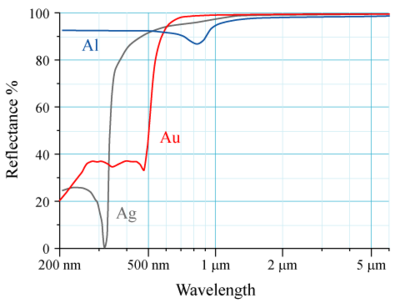In optics
Optics
Optics is the branch of physics which involves the behavior and properties of light, including its interactions with matter and the construction of instruments that use or detect it. Optics usually describes the behavior of visible, ultraviolet, and infrared light...

and photometry
Photometry (optics)
Photometry is the science of the measurement of light, in terms of its perceived brightness to the human eye. It is distinct from radiometry, which is the science of measurement of radiant energy in terms of absolute power; rather, in photometry, the radiant power at each wavelength is weighted by...

, reflectivity is the fraction of incident radiation reflected
Reflection (physics)
Reflection is the change in direction of a wavefront at an interface between two differentmedia so that the wavefront returns into the medium from which it originated. Common examples include the reflection of light, sound and water waves...

by a surface. In general it must be treated as a directional property that is a function of the reflected direction, the incident direction, and the incident wavelength. However it is also commonly averaged over the reflected hemisphere to give the hemispherical spectral reflectivity: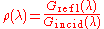where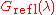and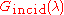are the reflected and incident spectral (per wavelength) intensity
Intensity (physics)
In physics, intensity is a measure of the energy flux, averaged over the period of the wave. The word "intensity" here is not synonymous with "strength", "amplitude", or "level", as it sometimes is in colloquial speech...

, respectively.

This can be further averaged over all wavelengths (in a given band of interest—say, optical) to give the total hemispherical reflectivity, or reflectance factor: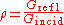## Reflectance

Reflectivity and reflectance generally refer to the fraction of incident electromagnetic power that is reflected at an interface, while the term "reflection coefficient" is used for the fraction of electric field reflected. The reflectance or reflectivity is thus the square of the magnitude of the reflection coefficient. The reflection coefficient can be expressed as a complex number
Complex number
A complex number is a number consisting of a real part and an imaginary part. Complex numbers extend the idea of the one-dimensional number line to the two-dimensional complex plane by using the number line for the real part and adding a vertical axis to plot the imaginary part...

as determined by the Fresnel equations for a single layer, whereas the reflectance (or reflectivity) is always a positive real number
Real number
In mathematics, a real number is a value that represents a quantity along a continuum, such as -5 , 4/3 , 8.6 , √2 and π...

.

According to the CIE (the International Commission on Illumination), reflectivity is distinguished from reflectance by the fact that reflectivity is a value that applies to thick reflecting objects. When reflection occurs from thin layers of material, internal reflection effects can cause the reflectance to vary with surface thickness. Reflectivity is the limit value of reflectance as the surface becomes thick; it is the intrinsic reflectance of the surface, hence irrespective of other parameters such as the reflectance of the rear surface. Another way to interpret this is that the reflectance is the fraction of electromagnetic power reflected from a specific sample, while reflectivity is a property of the material itself, which would be measured on a perfect machine if the material filled half of all space.

The reflectance spectrum or spectral reflectance curve is the plot of the reflectance as a function of wavelength.

## Surface type

Going back to the fact that reflectivity is a directional property, most surfaces can be divided into those that give specular reflection
Specular reflection
Specular reflection is the mirror-like reflection of light from a surface, in which light from a single incoming direction is reflected into a single outgoing direction...

and those that give diffuse reflection
Diffuse reflection
Diffuse reflection is the reflection of light from a surface such that an incident ray is reflected at many angles rather than at just one angle as in the case of specular reflection...

.
• For specular surfaces, such as glass or polished metal, reflectivity will be nearly zero at all angles except at the appropriate reflected angle - that is, reflected radiation will follow a different path from incident radiation for all cases other than radiation normal to the surface.

• For diffuse surfaces, such as matte white paint, reflectivity is uniform; radiation is reflected in all angles equally or near-equally. Such surfaces are said to be Lambertian
Lambertian reflectance
If a surface exhibits Lambertian reflectance, light falling on it is scattered such that the apparent brightness of the surface to an observer is the same regardless of the observer's angle of view. More technically, the surface luminance is isotropic...

.

Most real objects have some mixture of diffuse and specular reflective properties.

## Water reflectivity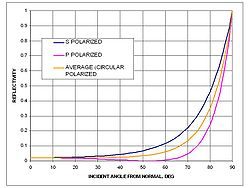Reflection occurs when light moves from a medium with one index of refraction into a second medium with a different index of refraction.

Specular reflection from a body of water is calculated by the Fresnel equations. Fresnel reflection is directional and therefore does not contribute significantly to albedo
Albedo
Albedo , or reflection coefficient, is the diffuse reflectivity or reflecting power of a surface. It is defined as the ratio of reflected radiation from the surface to incident radiation upon it...

which is primarily diffuse reflection.

A real water surface may be wavy. Reflectivity assuming a flat surface as given by the Fresnel equations
Fresnel equations
The Fresnel equations , deduced by Augustin-Jean Fresnel , describe the behaviour of light when moving between media of differing refractive indices...

can be adjusted to account for waviness
Waviness
Waviness is the measure of the more widely spaced component of surface texture. It is a broader view of roughness because it is more strictly defined as "the irregularities whose spacing is greater than the roughness sampling length"...

.

## Grating efficiency

The generalization of reflectance to a diffraction grating
Diffraction grating
In optics, a diffraction grating is an optical component with a periodic structure, which splits and diffracts light into several beams travelling in different directions. The directions of these beams depend on the spacing of the grating and the wavelength of the light so that the grating acts as...

, which disperses light by wavelength
Wavelength
In physics, the wavelength of a sinusoidal wave is the spatial period of the wave—the distance over which the wave's shape repeats.It is usually determined by considering the distance between consecutive corresponding points of the same phase, such as crests, troughs, or zero crossings, and is a...

, is called diffraction efficiency.

## Applications

Reflectivity is an important concept in the fields of optics
Optics
Optics is the branch of physics which involves the behavior and properties of light, including its interactions with matter and the construction of instruments that use or detect it. Optics usually describes the behavior of visible, ultraviolet, and infrared light...

, solar thermal energy
Solar thermal energy
Solar thermal energy is a technology for harnessing solar energy for thermal energy . Solar thermal collectors are classified by the United States Energy Information Administration as low-, medium-, or high-temperature collectors. Low-temperature collectors are flat plates generally used to heat...

, telecommunication
Telecommunication
Telecommunication is the transmission of information over significant distances to communicate. In earlier times, telecommunications involved the use of visual signals, such as beacons, smoke signals, semaphore telegraphs, signal flags, and optical heliographs, or audio messages via coded...

and radar
Radar
Radar is an object-detection system which uses radio waves to determine the range, altitude, direction, or speed of objects. It can be used to detect aircraft, ships, spacecraft, guided missiles, motor vehicles, weather formations, and terrain. The radar dish or antenna transmits pulses of radio...

.

## See also

• Bidirectional reflectance distribution function
Bidirectional reflectance distribution function
The bidirectional reflectance distribution function is a four-dimensional function that defines how light is reflected at an opaque surface...

• Emissivity
Emissivity
The emissivity of a material is the relative ability of its surface to emit energy by radiation. It is the ratio of energy radiated by a particular material to energy radiated by a black body at the same temperature...

• Lambert's cosine law
Lambert's cosine law
In optics, Lambert's cosine law says that the radiant intensity observed from a Lambertian surface or a Lambertian radiator is directly proportional to the cosine of the angle θ between the observer's line of sight and the surface normal. A Lambertian surface is also known as an ideal diffusely...

• Transmittance
Transmittance
In optics and spectroscopy, transmittance is the fraction of incident light at a specified wavelength that passes through a sample. A related term is absorptance, or absorption factor, which is the fraction of radiation absorbed by a sample at a specified wavelength...

• Sun path
Sun path
Sun path refers to the apparent significant seasonal-and-hourly positional changes of the sun as the Earth rotates, and orbits around the sun. The relative position of the sun is a major factor in the heat gain of buildings and in the performance of solar energy systems...

## External links

The source of this article is wikipedia, the free encyclopedia.  The text of this article is licensed under the GFDL.